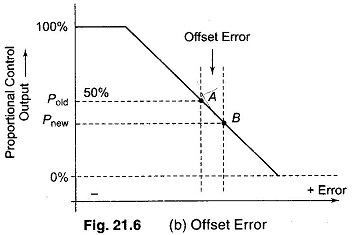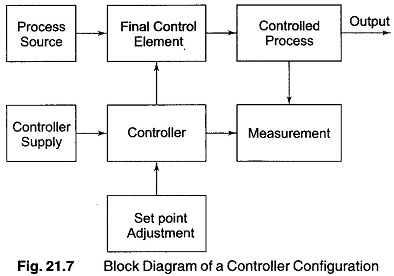## Define Offset Error:

Define Offset Error – Proportional control action is characterized by a permanent residual error in the operating point of the controlled variable when a change in load occurs. This error is called the OFFSET.

The offset can be reduced by selecting higher value of controller gain (KP) corresponding to narrow bandwidth.Figure 21.6 (b), shows how offset error occurs in a proportional control action. Let the system error be zero at nominal load with controller output P = 50% corresponding.

If, however, a transient error occurs, the system tends to adjust the controller output so that the point A (corresponding to zero error) is reached again. But for this to happen there must be change in the load system. This changed controller load produces a new value (Pnew) of the characteristic output, which gives rise to point B on the linear characteristic of the proportional controller. The permanent small difference between the percentage error values corresponding to point B and A is called the offset error of the f control.

### Basic Configuration Control

The primary requirement in most industrial processes is the control of typical process variables like temperature, pressure, flow or level, at reference value called set point. An instrument used to achieve this objective is called a controller.

It basically consists of a signal representing the value of a process variable, compares it with the set point value, and acts to minimize the difference between the two signals. A simplified block diagram of a controller configuration is shown in Fig. 21.7.The function of a controller is:

• receiving a signal corresponding to the measurement to be controlled,
• comparing that value to a reference set point value,
• determining the difference or error in the measurement value, and
• providing a controlled output.
Scroll to Top